# 终于，掘金也有人讲傅里叶变换了

## 二、写博客：傅里叶变换进行时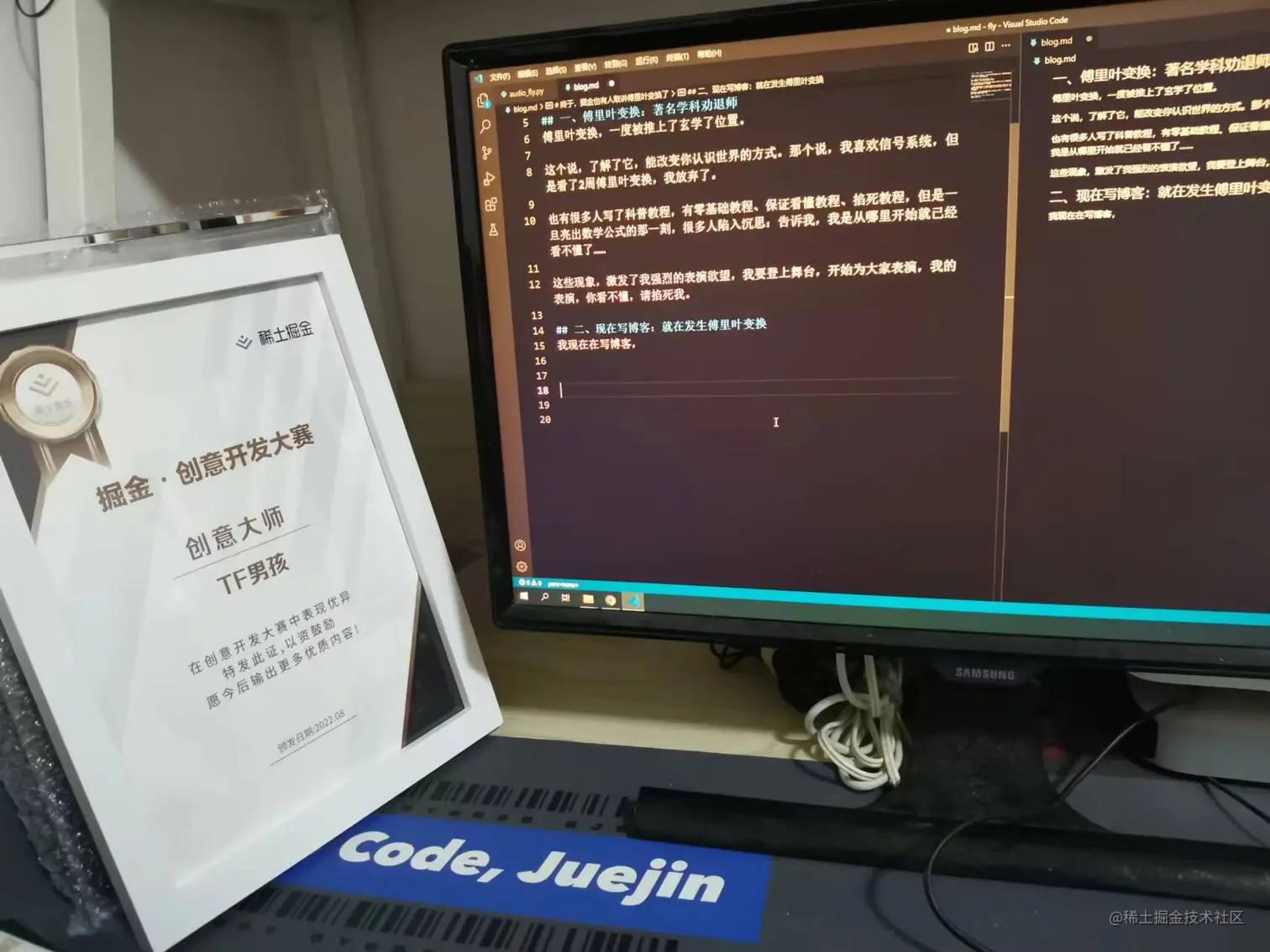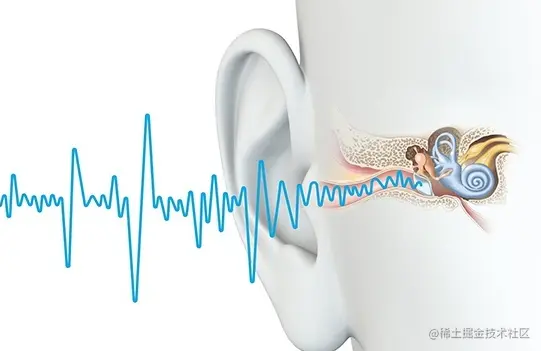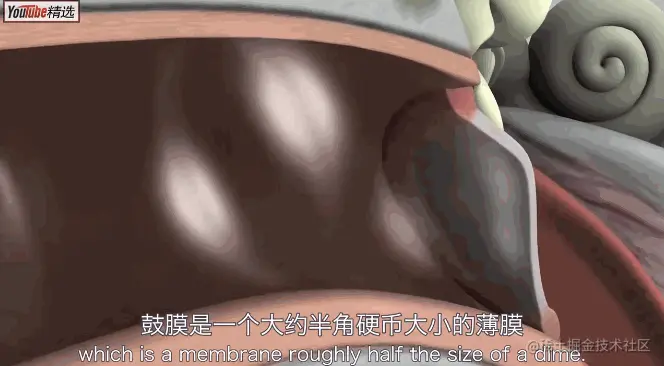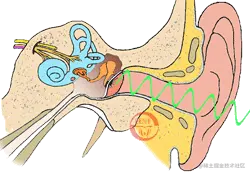## 三、描述一个波：都有独特的气质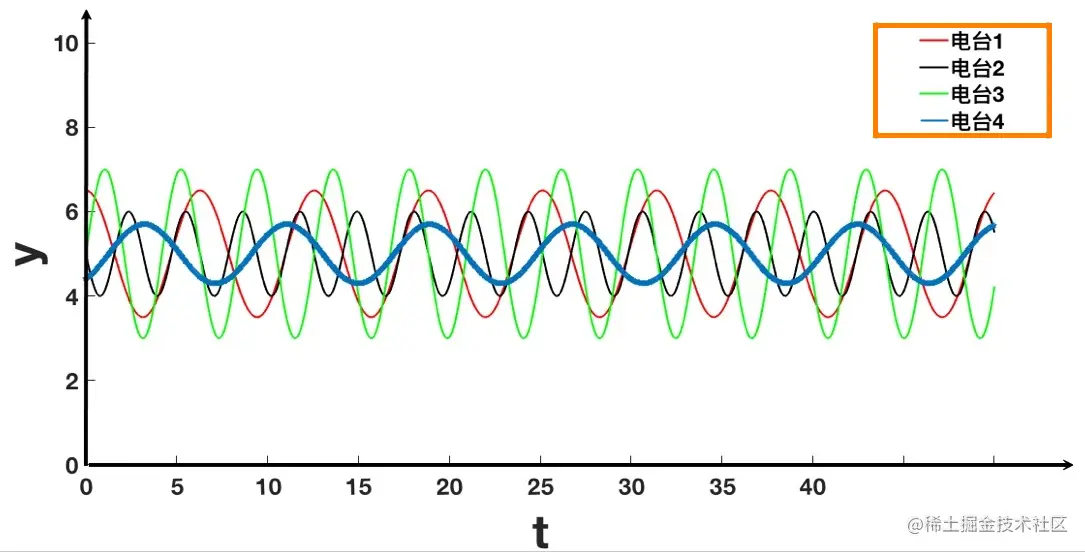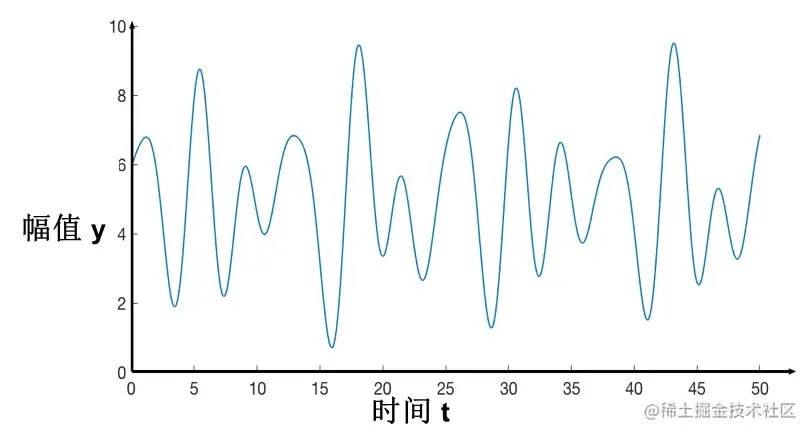### 3.1 声音的频率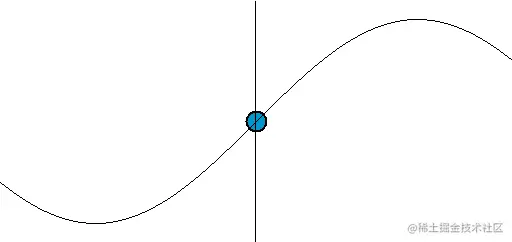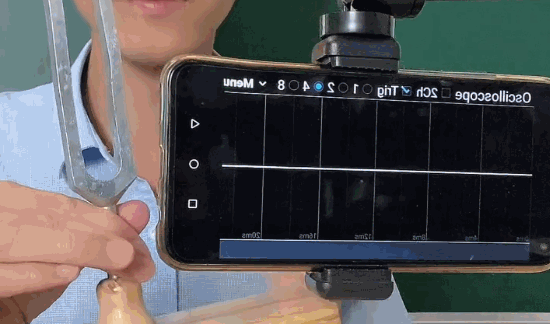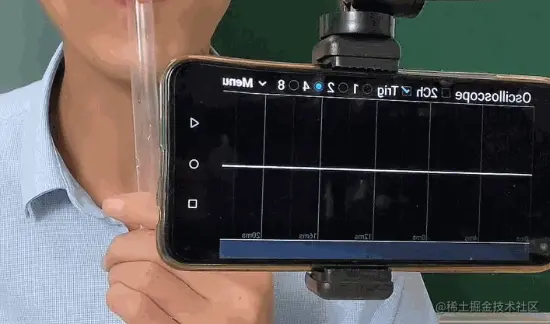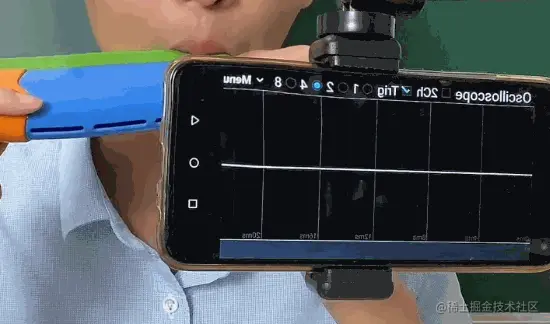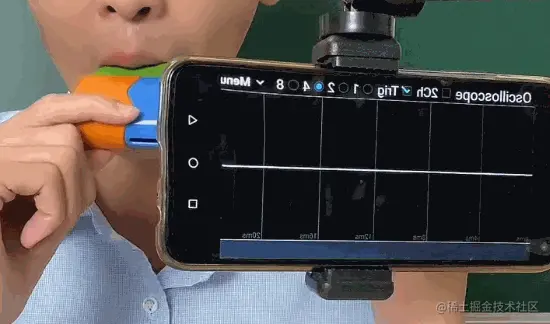### 3.2 声音的振幅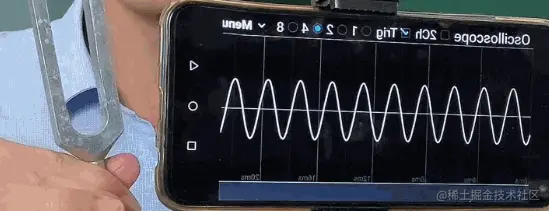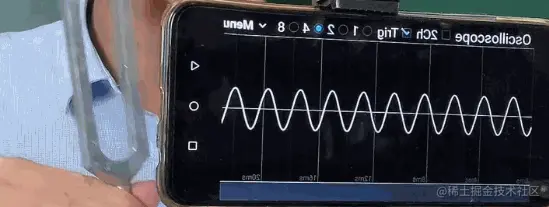## 四、说傅里叶变换吧：我不抗拒了！

### 4.1 时域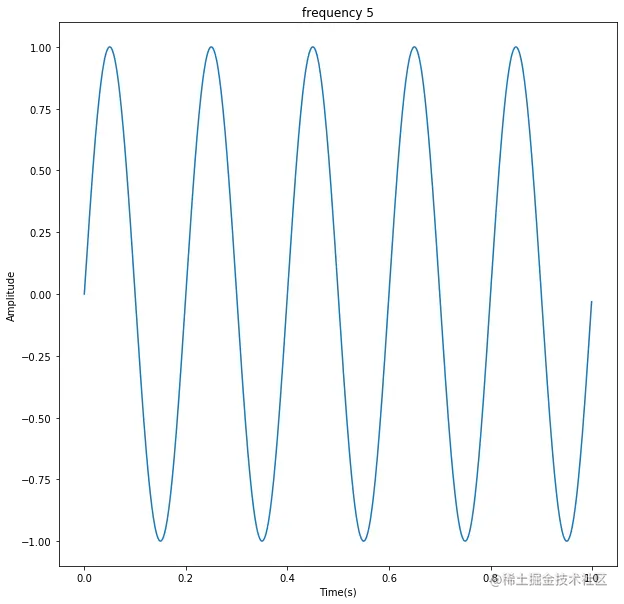### 4.2 频域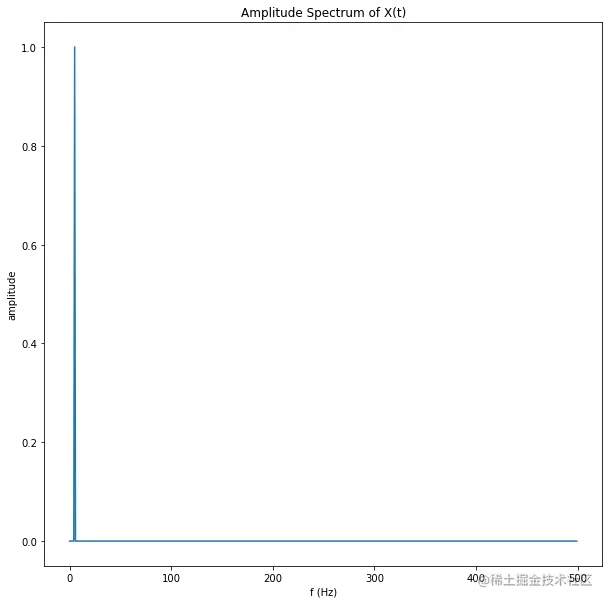### 4.3 波的合成和分解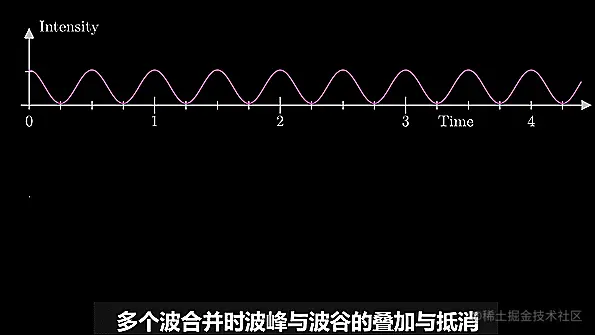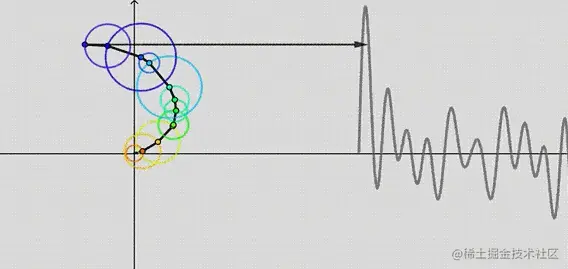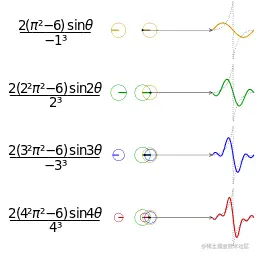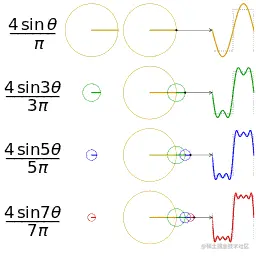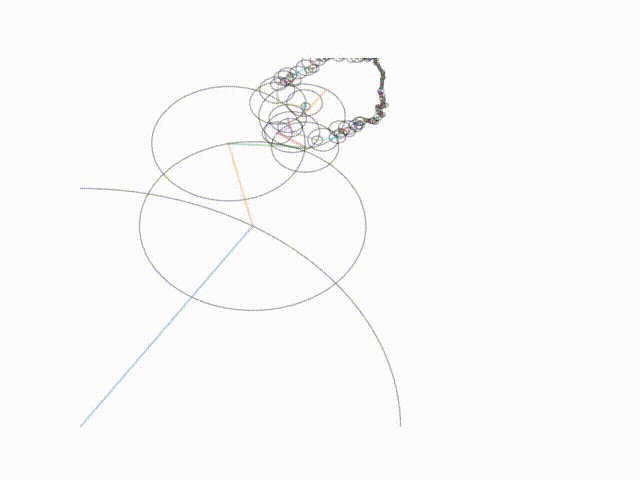### 4.4 时域拆解频域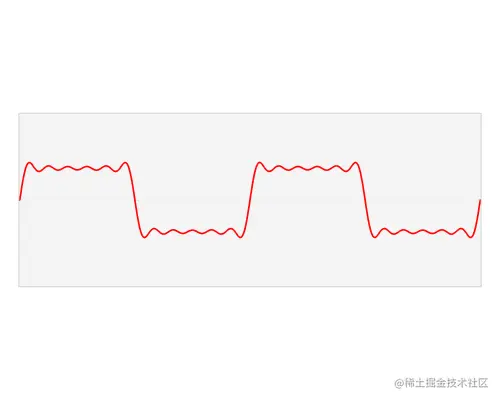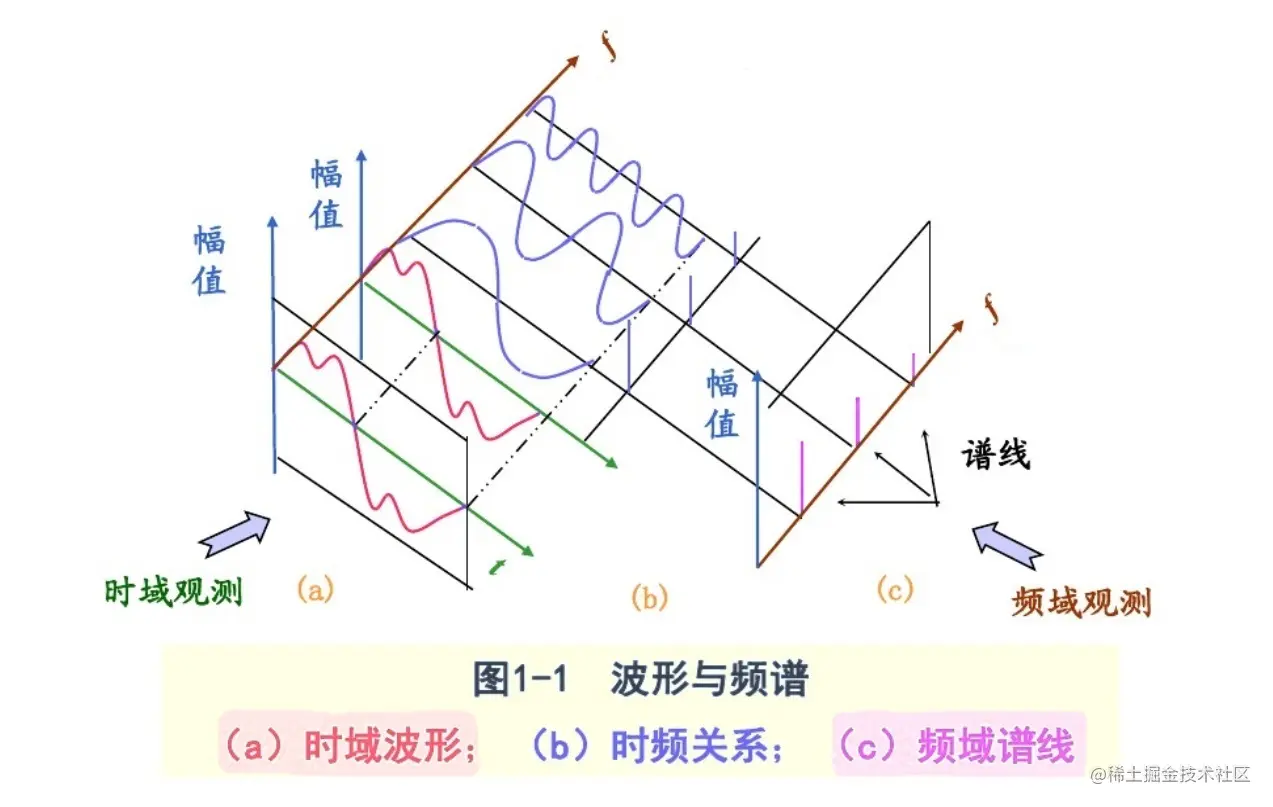### 4.5 频域复原时域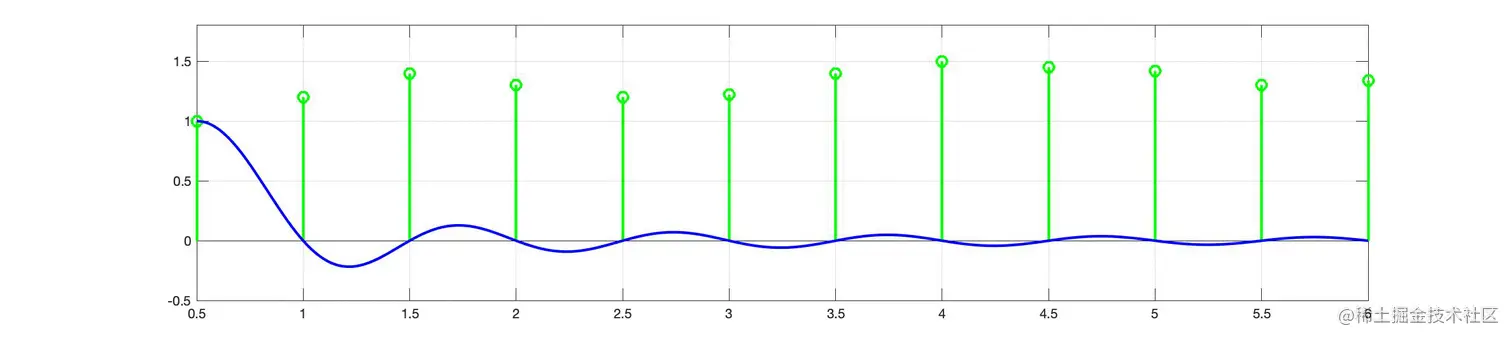## 五、傅里叶变换有什么用？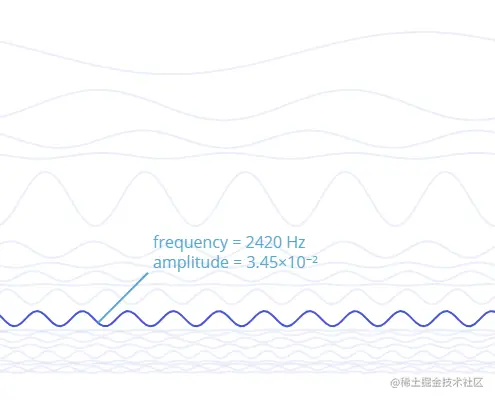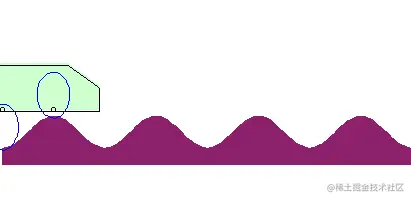## 六、代码实践

### 6.1 生成波形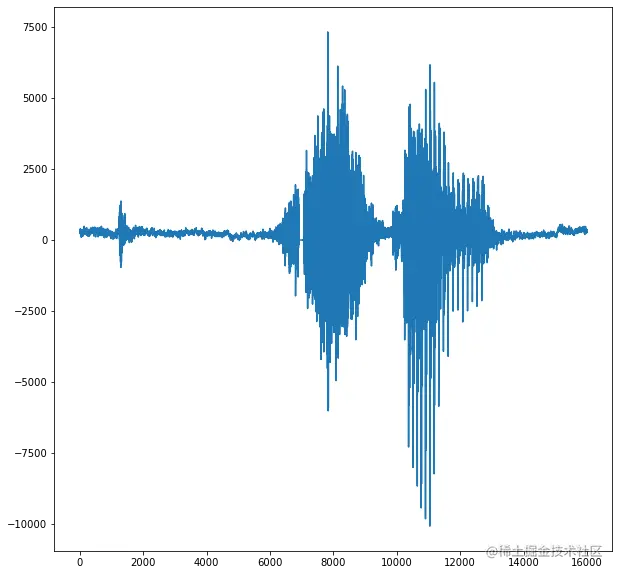``````from scipy.io import wavfile
import matplotlib.pyplot as plt
# 读取音频数据
# 采样率 fs, 音频数据 audio_wave
plt.plot(audio_wave) # 绘制音频数据
plt.show()
``````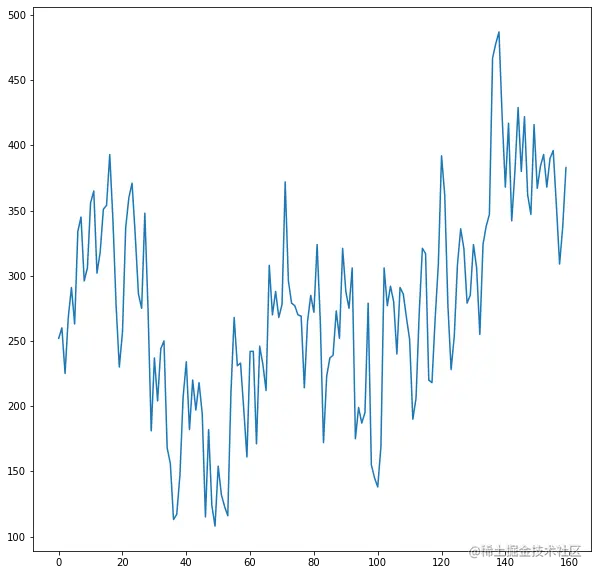#### 6.1.1 采样率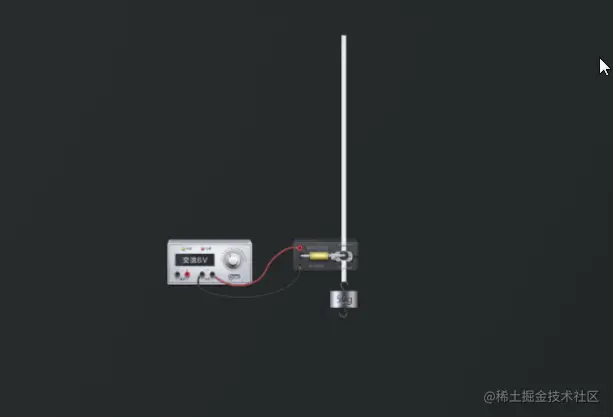60的采样率，能记录上信息吗？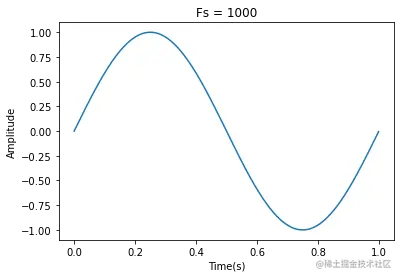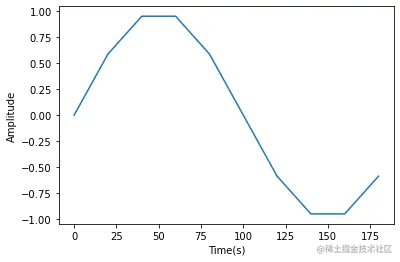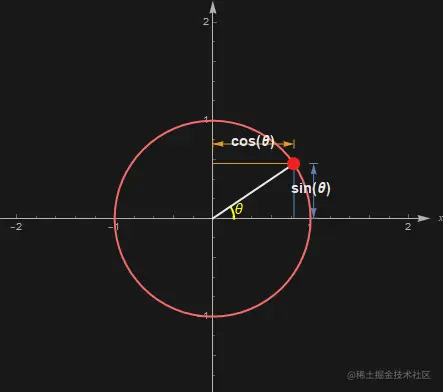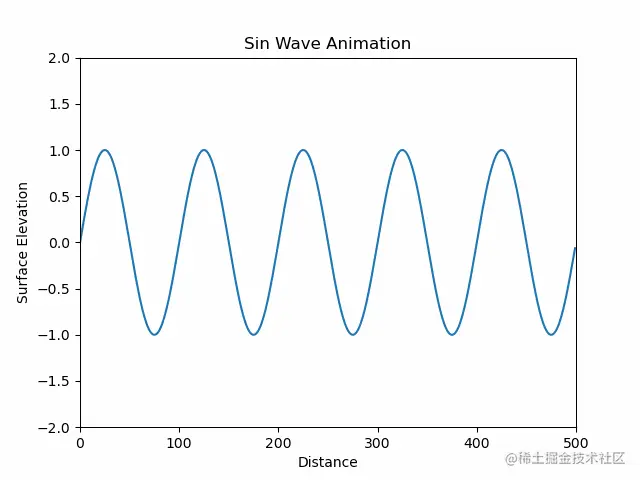#### 6.1.2 绘制波形

``````import numpy as np
#采样率，单位时间内采集多少次样本（1秒内记录1000次，采样率1kHz）
Fs = 1000
#采样周期，频率的倒数，相邻两点的时间间隔，1秒打10个点，采样周期就是0.1秒
T = 1/Fs
#信号长度，信号有多少个点
L = 1200
# 时间轴X轴的集合，这批信号每次采集的时间点
t = np.arange(L)*T
# 幅度值Y轴的集合，1200个点对应的Y轴的值
S = np.sin(2*np.pi*t)
``````

1秒内，t的值是`[0.000,0.001,0.002,……,1.0]`,把一个（完整周期）分成了1000份。对这1000个点求函数的值，也就是S = `[0,…0.5,…1,…0,…-0.5,…-1,…,0]`

``````import matplotlib.pyplot as plt
plt.plot(t[:1000], S[:1000])
plt.show()
``````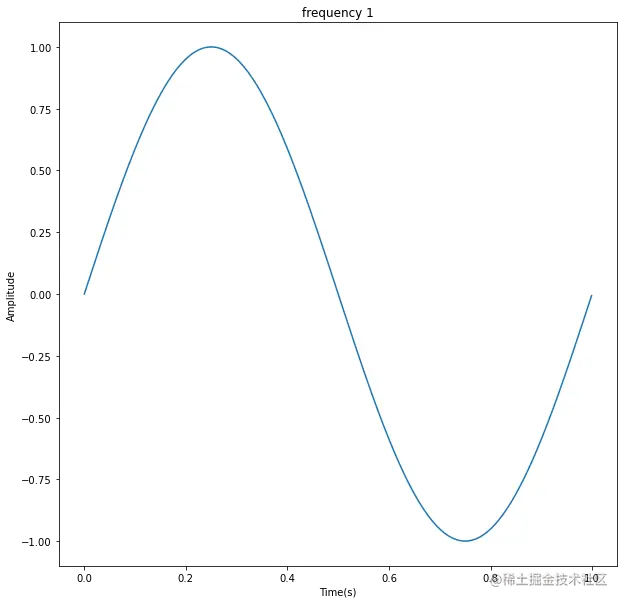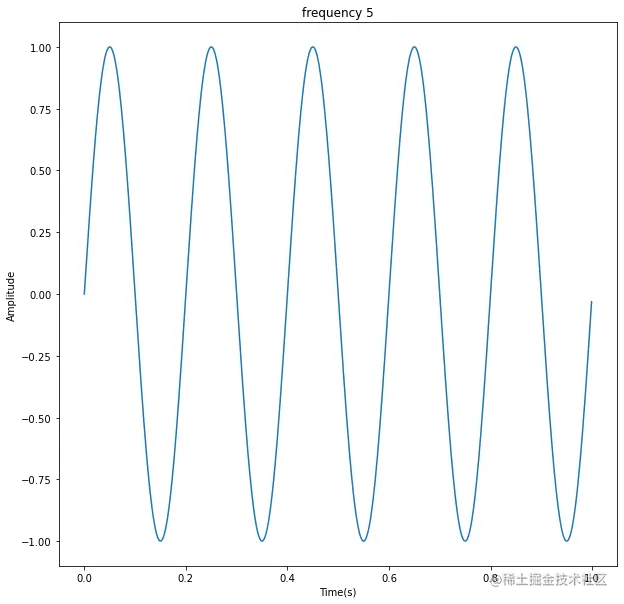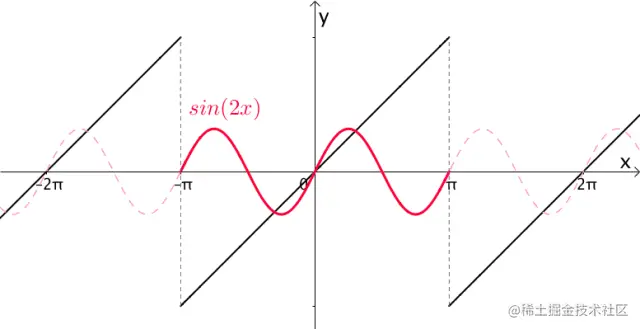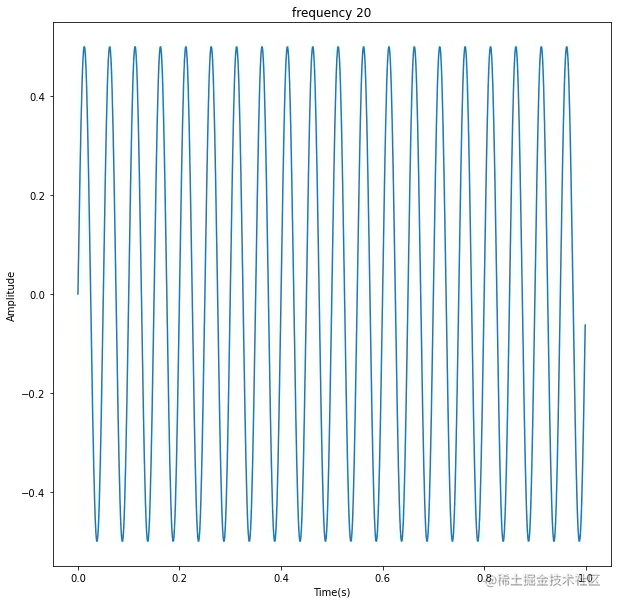``````S_5 = np.sin(2*np.pi*5*t)
S_20 = 0.5*np.sin(2*np.pi*20*t)
S_25 = S_5 + S_20
``````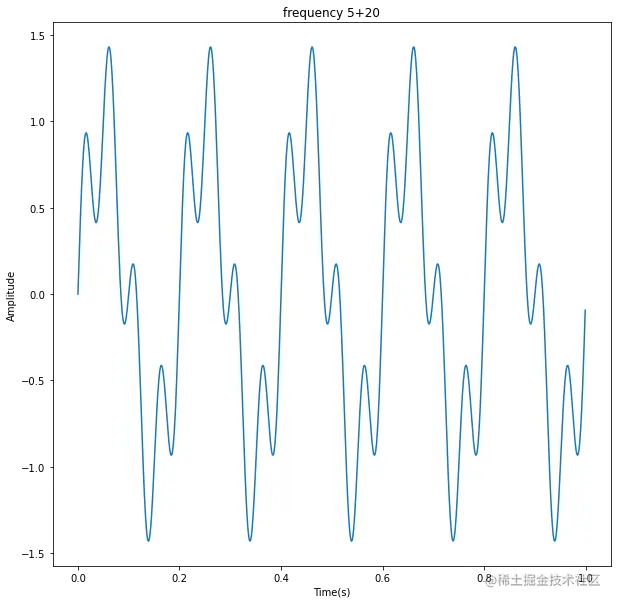### 6.2 傅里叶变换 FFT

python库`scipy.fftpack`中有`fft`，它就是专门处理傅里叶变换的。

``````from scipy.fftpack import fft
# 完成傅里叶变换
Y = fft(S_25)

# 把结果画出来
p2 = np.abs(Y)
p1 = np.abs(Y)[:int(L/2)]
# Fs、L是之前生成波形时的采样率和信号长度
f = np.arange(int(L/2))*Fs/L
plt.plot(f,2*p1/L)
plt.show()
``````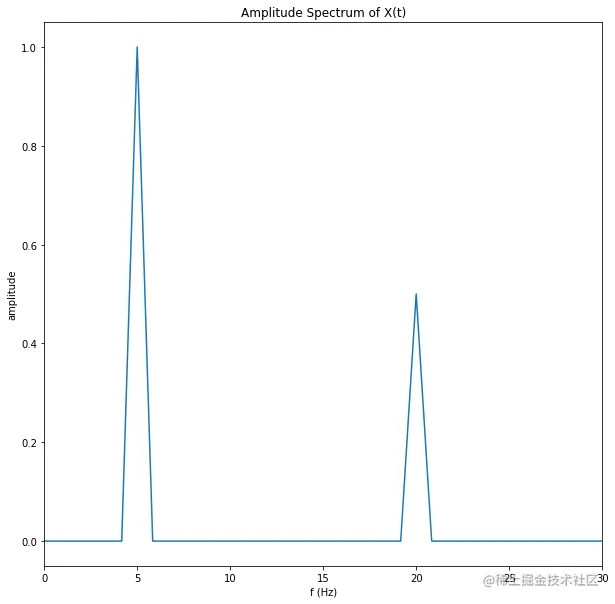``````S_20_5 = np.append(S_20, S_5)
``````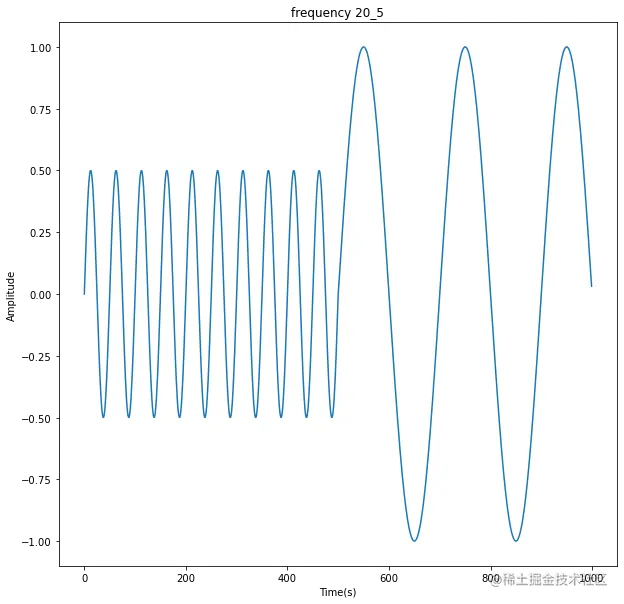### 6.3 短时傅里叶变换 STFT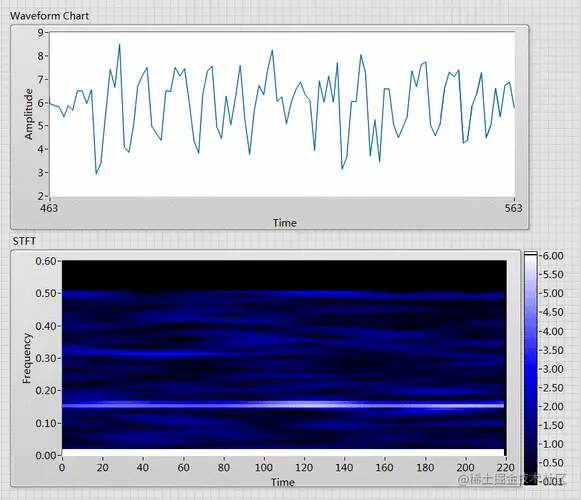``````from scipy.signal import stft

# 短时傅里叶变换
f, t, Z = stft(S_20_5, fs=1000)

# 把结果画出来
z = np.abs(Z)
plt.figure(figsize=(10,10))
plt.xlabel("author:handroid@126.com Time(s)")
plt.ylabel("frequency")
plt.ylim(ymin=0, ymax= 30)
plt.title("from https://juejin.cn/user/615370768790158")
c = plt.pcolormesh(t, f, z, cmap ='Greens', vmin = 0, vmax = 1)
plt.colorbar(c)
``````• 从纵坐标入手：这段信号，包含2段频率，一段频率是20，另一段频率是5。其中20频率段振幅较小，5频率段的振幅较大。
• 从横坐标入手：1.2s之前，只有高频信号；1.2s之后，高频消失，出现了低频信号，低频音量较大。
• 从振幅入手：声音较大（色值较重）的主要出现在后半段，且是低频信号发出，推测是男生发火了。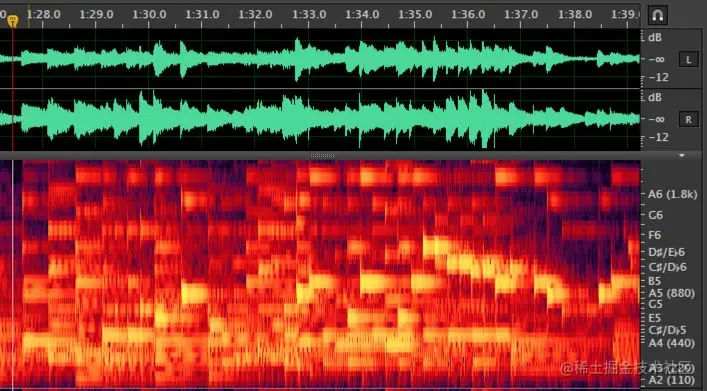## 七、保命条款•••••••